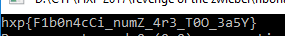Sunday, November 19, 2017

HXP CTF 2017 - "Fibonacci" Reversing 100 Writeup

Fibonacci - 100 pts + 6 bonus pts ( 45 solves ):
This binary is supposed to print the flag directly into the screen. However, it will take a very very long time to print the whole flag since the output is based on the calculation of fibonacci numbers recursively.
For each bit of the encoded flag (length = 33 stored at 00000000004007E0), the fibonacci number of that bit's position is calculated : this means that it will calculate fibonacci values for numbers from 0 to 263.
This is not all. Since the flag needs to be decoded, each call to the fibonacci sub-routine expects a pointer to a bit value which is XORed with a calculated bit from the resulting fibonacci number. Keep in mind that the fibonacci implementation is recursive, and thus we expects this boolean value to be XORed multiple times for greater numbers.
When the fibonacci sub-routine returns to the main function, the corresponding bit of the encrypted flag is XORed with the calculated bit value.

The solution that came in mind is to modify the fibonacci implementation so as to save both the calculated bit value and the resulting fibonacci number for a given number. So instead of recursing and re-calculating the fibonacci number of a previously calculated one (in a previous call for a previous bit of the flag), we simply load the result of the calculation and XOR the current output bit with the one we already saved.

The solution is implemented in the script below. All modifications done to the original function are commented.
Full script : here

We immediately get the flag when we run the program.